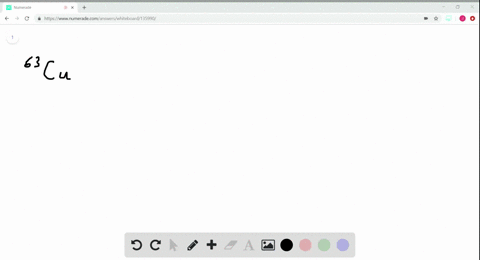Sign up for our free STEM online summer camps starting June 1st!View Summer Courses### Consider an atom of $^{63} \mathrm{Cu}$ (a) How m…

01:53Manhattan College

Need more help? Fill out this quick form to get professional live tutoring.

Get live tutoring
Problem 23

Consider an atom of 10 $\mathrm{B}$ . (a) How many protons, neutrons, and electrons does this atom contain? (b) What is the symbol of the atom obtained by adding one proton to $^{10} \mathrm{B} ?$ (c) What is the symbol of the atom obtained by adding one
neutron to 10 $\mathrm{B}$ ? (c) What is the symbol of the atom obtained by adding one
neutron to 10 $\mathrm{B} ?(\mathbf{d})$ Are either of the atoms obtained in parts (b) and (c) isotopes of 10 $\mathrm{B} ?$ If so which one?

a)
the number of protons is 5
the number of electrons is 5
the number of neutrons is 5
b)
$\frac{11}{6} \mathrm{C}$
c)
$\frac{11}{5} \mathrm{B}$
(d)
$\frac{11}{5} \mathrm{B}$

## Discussion

You must be signed in to discuss.

## Video Transcript

All right, guys, We're doing problem. 23 a chapter two. So we have our element and bore on 10. Uh, what's happening? Journal? Better be, uh, all right. Better now. How many protons? Neutrons, Electrons? Us to the outer contained. So you just go to your peer, I table and we see that Born has five protons. So you put a five down here. The number of protons, the same number of electrons. So it has five pro times, five electric. So and number of neutrons. We take this mass number and we subtract it by the atomic nick. Number five life. So tan minus five is five. So there are five protons, five neutrons and five electrons. Now, what is the symbol obtained by adding one? Proton too are born. So if we're adding one proton, that means we're increasing our atomic number. So then and and we'll have atomic number six and the atomic number six. That is carbon. Remember, if you add or remove a proton from an Adam, you're changing the kind of element that you have. No was assembled by adding one new trying to bore to erase this announce now is that Proton we're gonna add a new truck. So, neutron, all we're doing is increasing the mass of our of our element. So instead of 10 it's going to be 11 so it's gonna be bore on 11. So this the chemical symbol now are either of the Adams obtained in Parts B and C isotopes of boron on 10? If so, which one? So the one that's an isotope of boring 10. That's going to be this boring 11. Remember, wouldn't isotope is isotopes are two Adams that the same number of protons, but different number of nutrients. They're the same element, but they have different new numbers of neutrons. So these were both born. They both have five pro times, but because they have different number of nutrients, they're different elements.